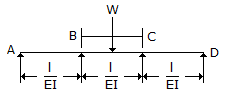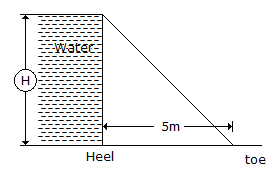# Civil Engineering - UPSC Civil Service Exam Questions

26.

For a symmetrical continuous beam as shown in the figure given above, which one of the following is correct in respect of distribution factors at B ?A. δBA : δBC = 3:4 B. δBA : δBC = 1:2 C. δBA : δBC = 3:2 D. δBA : δBC = 3:8

Explanation:

No answer description available for this question. Let us discuss.

27.

For the retaining wall shown in the given figure, if the stress at the heel is zero, then the maximum storage 'H' will be specific gravity of the material of the wall = 2.25A. 7.5 m B. 5 m C. 4 m D. 3 m

Explanation:

No answer description available for this question. Let us discuss.

28.

A short column of external diameter D1 and internal diameter D2 carries an external load W. For no-tension condition, the eccentricity will be :

 A. (D12 - D22)/8D2 B. (D21 - D22)/8D2 C. (D12 - D22)/8D1 D. (D1 - D2)2 /8D1

Explanation:

No answer description available for this question. Let us discuss.

29.

The following four hydrological features have to be estimated or taken as inputs before one can compute the flood hydrograph at any catchment outlet:
1. Unit hydrograph
2. Rainfall hydrograph
3. Infiltration index
4. Base flow
The correct order in which they have to be employed in the computations is

 A. 1, 2, 3, 4 B. 2, 1, 4, 3 C. 2, 3, 1, 4 D. 4, 1, 3, 2

Explanation:

No answer description available for this question. Let us discuss.

30.

Which one of the following statements is correct ?
A forebay in a hydel system is provided at the junction of :

 A. the power channel and the tail race channel B. the tail race channel and the penstock C. the penstock and the turbine D. the power channel and the penstock